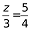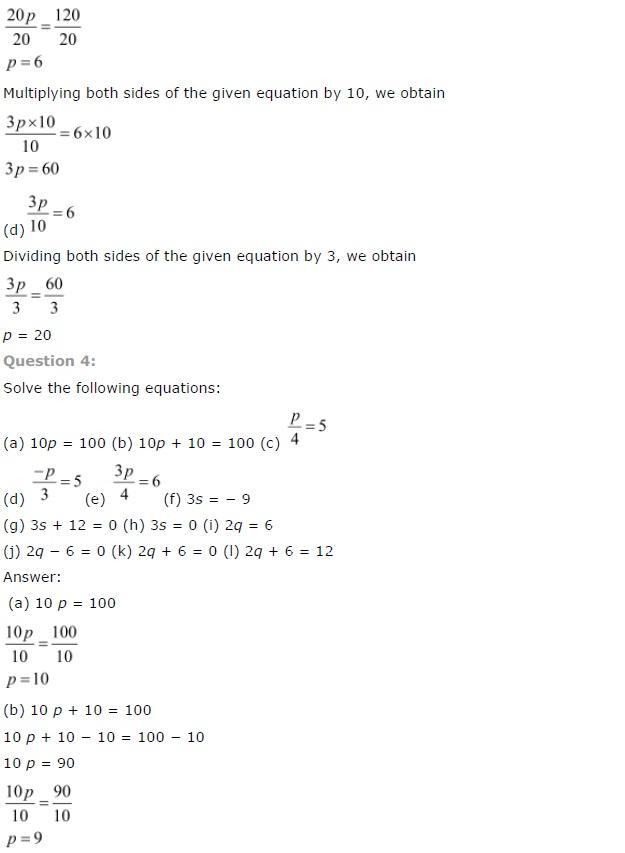# NCERT Solutions class 7 Maths Chapter-4 Exercise 4.2

NCERT Solutions Class-7 Maths chapter-4 Linear equation  Exercise-4.2 is prepared by academic team of Physics Wallah all the questions of NCERT text book are solved step by step with proper and detail solutions explaining each and every questions . For More and additional questions of CBSE class 7 maths you can go to class 7 maths sections. NCERT class 7 Maths Solutions  is the best way to enhanced your mathematics skill. And Physics Wallah practice worksheet & question bank will help you a lot.

## NCERT Solutions class 7 Maths Chapter-4 Linear equation

### Solutions of Chapter Linear equation  Exercise-4.2

Question 1:
Give first the step you will use to separate the variable and then solve the equation:
(a) x – 1 = 0
(b) x + 1 = 0
(c) x – 1 = 5
(d) x + 6 = 2
(e) y – 4 = – 7
(f) y – 4 = 4
(g) y + 4 = 4
(h) y + 4 = – 4

Answer: (a) x – 1 = 0

Solution: We have to separate the variables
Thus, x – 1 = 0
Now,
Adding 1 on both side of the equation, We get,
x – 1 + 1 = 0 + 1
x = 1
(b) x + 1 = 0

Solution: Here, we have to separate the variables
We have,
x + 1 = 0
Now,
Subtracting 1 on both side of the equation,We get,
x – 1 + 1 = 0 – 1
x = -1
(c) x – 1 = 5

Solution: Here, we have to separate the variables
We have,
x – 1 = 5
Now,Adding 1 on both side of the equation,We get,
x – 1 + 1 = 5 + 1
x = 6
(d) x + 6 = 2

Solution: Here, we have to separate the variables
We have,
x + 6 = 2
Now, subtracting 6 on both side of the equation,
We get,
x + 6 - 6 = 2 – 6
x = -4
(e)y – 4 = – 7

Solution: Here, we have to separate the variables
We have,
y – 4 = -7
Now,
Adding 4 on both side of the equation,
We get,
x – 4 + 4 = -7 + 4
x = -3
(f) y – 4 = 4

Solution: Here, we have to separate the variables
We have,
y – 4 = 4
Now,Adding 4 on both side of the equation,We get,
x – 4 + 4 = 4 + 4
x = 8
(g) y + 4 = 4

Solution: Here, we have to separate the variables
Thus,
We have, y + 4 = 4
Now,
Subtracting 4 on both side of the equation, We get,
x + 4 - 4 = 4 - 4
x = 0
(h )y + 4 = – 4

Solution: Here,We have to separate the variables
Thus,We have,
y + 4 = -4
Now,
Subtracting 4 on both side of the equation,We get,
x + 4 – 4 = -4 – 4
x = -8

Question 2:
Check whether the value given in the brackets is a solution to the given equation or not:
(a)3l = 42
(b )b/2 = 6
(c)p/7 = 4
(f)(h)20t = -10

The given parts of the question are solved below:
Here,
Thus,
Dividing both sides by 3, we get, l=423
We have to separate the variables
Multiplying both sides by 2, we get,
b = 12
(c) p/7 = 4
We have, p7=4
p/7×7 = 4 × 7